## Introduction

The previous set of notes discussed how to implement the ListADT interface using an array to store the items in the list. Here we discuss how to implement the ListADT interface using a linked list to store the items. However, before talking about linked lists, we will review the difference between primitive and non-primitive types in Java.

## Java Types

Java has two "categories" of types:

1. primitive types: short, int, long, float, double, boolean, char, and byte
2. reference types: arrays and classes

When you declare a variable with a primitive type, you get enough space to hold a value of that type. Here's some code involving a primitive type and the corresponding conceptual picture: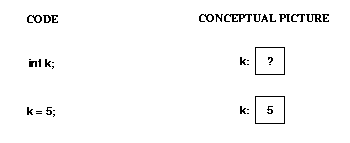When you declare a variable with a reference type, you get space for a reference (or pointer) to that type, not for the type itself. You must use "new" to get space for the type itself. This is illustrated below.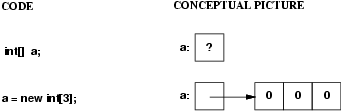Remember that class objects are also reference types. For example, if you declare a variable of type List, you only get space for a pointer to a list; no actual list exists until you use "new". This is illustrated below, assuming the array implementation of lists and assuming that the ArrayList constructor initializes the items array to be of size 3. Note that because it is an array of Objects, each array element is (automatically) initialized to null (shown using a diagonal line in the picture).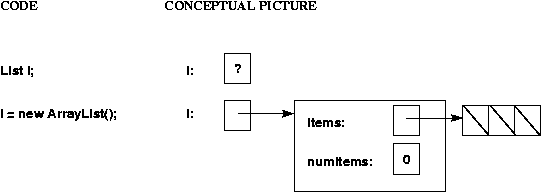An important consequence of the fact that non-primitive types are really pointers is that assigning from one variable to another can cause aliasing (two different names refer to the same object). For example: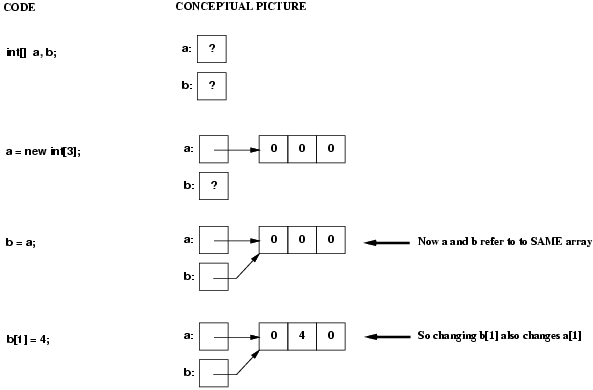Note that in this example, the assignment to b changed not only that value, but also the value in a (because a and b were pointing to the same array)! However, an assignment to b itself (not to an element of the array pointed to by b) has no effect on a: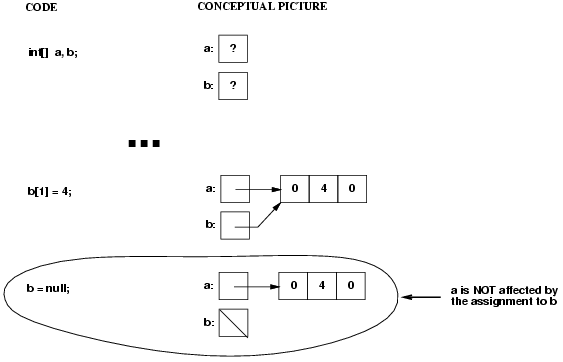A similar situation arises when a non-primitive value is passed as an argument to a method. For example, consider the following 4 statements and the definition of method changeArray:

```1.   int[] a = new int;
2.   a = 6;
3.   changeArray(a);
4.   System.out.print(a);

5.   public static void changeArray(int[] x) {
6.     x = 10;
7.     x = new int;
8.   }
```

The picture below illustrates what happens when this code executes.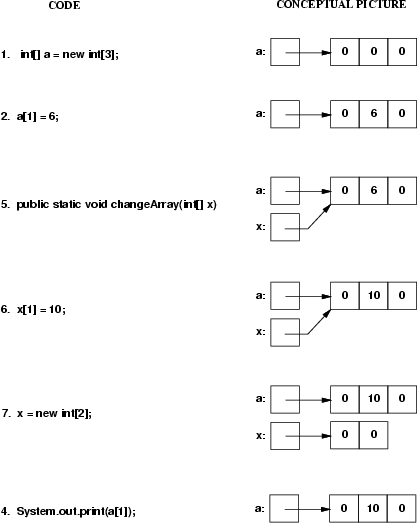Note that the method call causes A and X to be aliases (they both point to the same array). Therefore, the assignment X = 10 changes both X and A. However, the assignment X = new int only changes X, not A, so when the call to changeArray finishes, the value of A is still 10, not 0.

TEST YOURSELF #1

For each line of the code shown below, draw the corresponding conceptual picture.

```int [] x = new int;
x = 1;
int y = new int;
y = 2;
y = x;
y = 3;
```

solution

Here's a conceptual picture of a linked list containing N items, pointed to by a variable named l: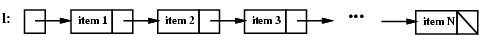Note that a linked list consists of one or more nodes. Each node contains some data (in this example, item 1, item 2, etc,) and a pointer. For each node other than the last one, the pointer points to the next node in the list. For the last node, the pointer is null (indicated in the example using a diagonal line). To implement linked lists in Java, we will define a Listnode class, to be used to represent the individual nodes of the list.

```public class Listnode<E> {
//*** fields ***
private E data;
private Listnode<E> next;

//*** constructors ***
// 2 constructors
public Listnode(E d) {
this(d, null);
}

public Listnode(E d, Listnode n) {
data = d;
next = n;
}

//*** methods ***
public E getData() {
return data;
}

public Listnode<E> getNext() {
return next;
}

// modify fields
public void setData(E d) {
data = d;
}

public void setNext(Listnode<E> n) {
next = n;
}
}
```

Note that the next field of a Listnode<E> is itself of type Listnode<E>. That works because in Java, every non-primitive type is really a pointer; so a Listnode<E> object is really a pointer that is either null or points to a piece of storage (allocated at runtime) that consists of two fields named data and next.

To understand this better, consider writing code to create a linked list of Strings with two nodes, containing "ant" and "bat", respectively, pointed to by a variable named l. First we need to declare variable l; here's the declaration together with a picture showing what we have so far: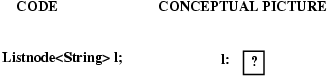To make l point to the first node of the list, we need to use new to allocate space for that node. We want its data field to contain "ant" and (for now) we don't care about its next field, so we'll use the 1-argument Listnode constructor (which sets the next field to null):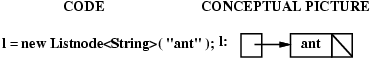To add the second node to the end of the list we need to create the new node (with "bat" in its data field and null in its next field) and we need to set the next field of the first node to point to the new one: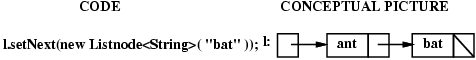TEST YOURSELF #2

Assume that the list shown above (with nodes "ant" and "bat") has been created.

Question 1: Write code to change the contents of the second node's data field from "bat" to "cat".

Question 2: Write code to insert a new node with "rat" in its data field between the two existing nodes.

• Adding a node after a given node in the list.
• Removing a given node from the list.

Assume that we are given:

1. n, (a pointer to) a node in a list (i.e., n is a Listnode), and
2. newdat, the data to be stored in a new node

and that the goal is to add a new node containing newdat immediately after n. To do this we must perform the following steps:

Step 1: create the new node using the given data

1. make the new node's next field point to whatever n's next field was pointing to
2. make n's next field point to the new node.

Here's the conceptual picture: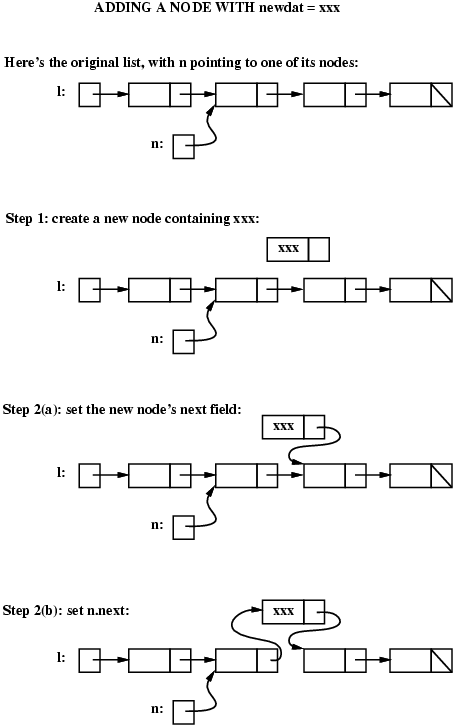And here's the code:

```Listnode<String> tmp = new Listnode<String>(newdat);    // Step 1
tmp.setNext( n.getNext() );                             // Step 2(a)
n.setNext( tmp );                                       // Step 2(b)
```

Note that it is vital to first copy the value of n's next field into tmp's next field (step 2(a)) before setting n's next field to point to the new node (step 2(b)). If we set n's next field first, we would lose our only pointer to the rest of the list after node n!

Also note that, in order to follow the steps shown in the picture above, we needed to use variable tmp to create the new node (in the picture, step 1 shows the new node just "floating" there, but that isn't possible -- we need to have some variable point to it so that we can set its next field and so that we can set n's next field to point to it). However, we could in fact accomplish steps 1 and 2 with a single statement that creates the new node, fills in its data and next fields, and sets n's next field to point to the new node! Here is that amazing statement:

```n.setNext( new Listnode<String>(newdat, n.getNext()) );  // steps 1, 2(a), and 2(b)
```

TEST YOURSELF #3

Draw pictures like the ones given above, to illustrate what happens when node n is the last node in the list. Does the statement

`n.setNext( new Listnode<String>(newdat, n.getNext()) );`

still work correctly?

Now consider the worst-case running time for this add operation. Whether we use the single statement or the list of three statements, we are really doing the same thing:

1. Using new to allocate space for a new node (start step 1).
2. Initializing the new node's data and next fields (finish step 1 + step 2(a)).
3. Changing the value of n's next field (step 2(b)).

We will assume that storage allocation via new takes constant time. Setting the values of the three fields also takes constant time, so the whole operation is a constant-time (O(1)) operation. In particular, the time required to add a new node immediately after a given node is independent of the number of nodes already in the list.

### Removing a node

To remove a given node n from a linked list, we need to change the next field of the node that comes immediately before n in the list to point to whatever n's next field was pointing to. Here's the conceptual picture: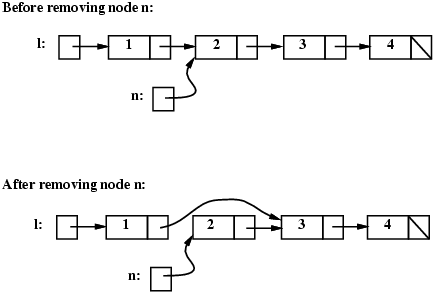Note that the fact that n's next field is still pointing to a node in the list doesn't matter -- n has been removed from the list, because it cannot be reached from l. It should be clear that in order to implement the remove operation, we first need to have a pointer to the node before node n (because that node's next field has to be changed). The only way to get to that node is to start at the beginning of the list. We want to keep moving along the list as long as the current node's next field is not pointing to node n. Here's the appropriate code:

```Listnode<String> tmp = l;
while (tmp.getNext() != n) {  // find the node before n
tmp = tmp.getNext();
}```

Note that this kind of code (moving along a list until some condition holds) is very common. For example, similar code would be used to implement a lookup operation on a linked list (an operation that determines whether there is a node in the list that contains a given piece of data).

Note also that there is one case when the code given above will not work. When n is the very first node in the list, the picture is like this: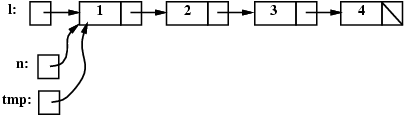In this case, the test (tmp.getNext() != n) will always be false and eventually we will "fall off the end" of the list (i.e., tmp will become null and we will get a runtime error when we try to dereference a null pointer). We will take care of that case in a minute; first, assuming that n is not the first node in the list, here's the code that removes n from the list:

```Listnode<String> tmp = l;
while (tmp.getNext() != n) {  // find the node before n
tmp = tmp.getNext();
}
tmp.setNext( n.getNext() );   // remove n from the linked list```

How can we test whether n is the first node in the list and what should we do in that case? If n is the first node, then l will be pointing to it, so we can test whether l == n. The following before and after pictures illustrate removing node n when it is the first node in the list: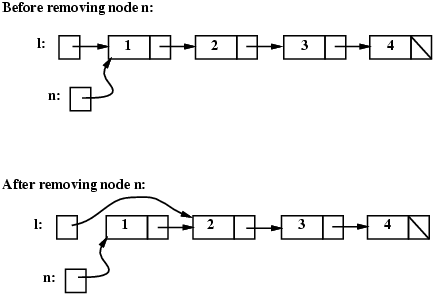```if (l == n) {
// special case: n is the first node in the list
l = n.getNext();
} else {
// general case: find the node before n, then "unlink" n
Listnode<String> tmp = l;
while (tmp.getNext() != n) {  // find the node before n
tmp = tmp.getNext();
}
tmp.setNext(n.getNext());
}```

What is the worst-case running time for this remove operation? If node n is the first node in the list, then we simply change one field (l's next field). However, in the general case, we must traverse the list to find the node before n, and in the worst case (when n is the last node in the list), this requires time proportional to the number of nodes in the list. Once the node before n is found, the remove operation involves just one assignment (to the next field of that node), which takes constant time. So the worst-case time running time for this operation on a list with N nodes is O(N).

There is an alternative to writing special-case code to handle removing the first node in a list. That alternative is to use a header node: a dummy node at the front of the list that is there only to reduce the need for special-case code in the linked-list operations. For example, the picture below shows how the list "ant", "bat", "cat", would be represented using a linked list without and with a header node: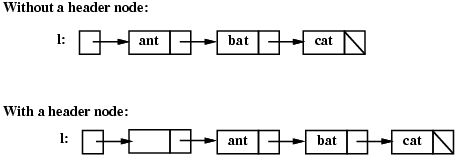Note that if your linked lists do include a header node, there is no need for the special case code given above for the remove operation; node n can never be the first node in the list, so there is no need to check for that case. Similarly, having a header node can simplify the code that adds a node before a given node n.

Note that if you do decide to use a header node, you must remember to initialize an empty list to contain one (dummy) node, you must remember not to include the header node in the count of "real" nodes in the list (e.g., if you implement a size operation), and you must remember to ignore the header node in operations like contains.

Now let's consider how to implement our ListADT interface using linked list instead of arrays; i.e., how to implement a scaled-down LinkedList class. Remember, we only want to change the implementation (the "internal" part of the List abstract data type), not the interface (the "external" part of the abstract data type). That means that the signatures of the public methods for the LinkedList class will be the same as the ones for the SimpleArrayList class (and the ListADT interface) and the descriptions of what those methods do will also be the same. The only things that will change are how the list is represented and how the methods are implemented.

Look back at the definition of the SimpleArrayList class in the second set of notes and think about which fields and/or methods need to be changed before reading any further.

Clearly, the type of the items field needs to change, since the items will no longer be stored in an array; instead, the items will be stored in a linked list. Since having a header node simplifies the add and remove operations, we will assume that the linked list has a header node. Here's new declaration for the items field:

`private Listnode<E> items;  // pointer to the header node of the list of items`

Given this declaration for the items field, let's think again about the three List methods that were discussed assuming the array implementation:

1. The version of add that adds to the end of the list.
2. The version of add that adds to a given position in the list.
3. The constructor.

### add (to end of list)

Recall that the first version of method add adds a given value to the end of the list. We have already discussed how to add a new node to a linked list following a given node. The only question is how best to handle adding a new node at the end of the list. A straightforward approach would be to traverse the list, looking for the last node (i.e., use a variable tmp as was done above in the code that looked for the node before node n). Once the last node is found, the new node can be inserted immediately after it.

The disadvantage of this approach is that it requires O(N) time to add a node to the end of a list with N items. An alternative is to add a lastNode field (often called a tail pointer) to the LinkedList class and to implement the methods that modify the linked list so that lastNode always points to the last node in the linked list (which will be the header node if the list is empty). There is more opportunity for error (since several methods will need to ensure that the lastNode field is kept up to date), but the use of the lastNode field will mean that the worst-case running time for this version of add is always O(1) and that will be important for applications that frequently add to the end of a list (which is often a common operation).

Here's a picture of the "ant, bat, cat" list, when the implementation includes a lastNode pointer: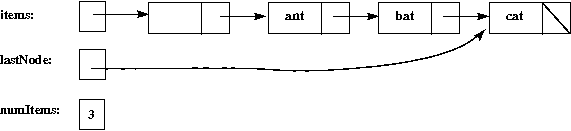TEST YOURSELF #4

Write the "add at the end" method (assuming that the LinkedList class includes both a header node and a lastNode field).

### add (at a given position)

As discussed above for the "add to the end" method, we already know how to add a node to a linked list after a given node. So to add a node at position pos, we just need to find the previous node in the list. Since we're assuming that our LinkedList class is implemented with a header node, there will always be such a node (i.e., we don't need any special-case code when we're asked to add a node at the beginning of the list).

TEST YOURSELF #5

Write the "add at a given position" method (assuming that the LinkedList class includes both a header node and a lastNode field).

The LinkedList constructor needs to initialize the three fields:

1. Listnode<E> items (the pointer to the header node)
2. Listnode<E> lastNode (the pointer to the last node in the list)
3. int numItems

so that the list is empty. An empty list is one that has just a header node, pointed to by both items and lastNode. As for the array implementation, numItems should be set to zero.

TEST YOURSELF #6

Write the constructor.

## Comparison: Lists via Arrays versus via Linked Lists

When comparing the List implementations using linked lists and using arrays, we should consider:

• space requirements
• time requirements
• ease of implementation

• In the linked-list implementation, one pointer must be stored for every item in the list, while the array stores only the items themselves.
• On the other hand, the space used for a linked list is always proportional to the number of items in the list. This is not necessarily true for the array implementation as described: if a lot of items are added to a list and then removed, the size of the array can be arbitrarily greater than the number of items in the list. However, we could fix this problem by modifying the remove operation to shrink the array when it becomes too empty.

In terms of time:

• The expandArray method discussed in class requires O(N) for adding an item to the end of a list because when the List becomes full, we copy the entire array's contents into a new bigger array. Consider an alternate implementation using a "shadow array", where instead of waiting until the original array is completely full, create a second larger array after some threshold of fullness. Say when first array is 75% full, create a second array of double the size. As new items are added to first array, copy one item from the first array into the second array along with the new item. Maintain the required length indicator variables to track size of first array and size of second array. Thus the add operation is still O(1) instead of O(N) cost. Get rid of the first array once second array is completely updated. It is also O(1) for the linked-list implementation as long as we have a lastNode field.
• Adding an item at a given position requires O(N) worst-case time for the array implementation, because existing items need to be moved. The operation is also O(N) in the worst case for the linked-list implementation, because we have to find the node currently in that position. So this operation is worst-case O(N) for both implementations. However, it is worth noting that for the array implementation adding closer to the beginning of the list is worst and adding toward the end of the list is best, while it is the other way around for the linked-list implementation.
• The get operation is O(1) for the array implementation and worst-case O(N) for the linked-list implementation.

In terms of ease of implementation, straightforward implementations of both the array and linked-list versions seem reasonably easy. However, the methods for the linked-list version seem to require more special cases.

TEST YOURSELF #7

Assume that lists are implemented using linked lists with header nodes and pointers to the last node in the list. How much time is required (using Big-O notation) to remove the first item from a list? to remove the last item from a list? How do these times compare to the times required for the same operations when the list is implemented using an array?

There are several variations on the basic idea of linked lists. Here we will discuss two of them:

Recall that, given (only) a pointer to a node n in a linked list with N nodes, removing node n takes time O(N) in the worst case, because it is necessary to traverse the list looking for the node just before n. One way to fix this problem is to require two pointers: a pointer to the node to be removed and also a pointer to the node just before that one.

Another way to fix the problem is to use a doubly linked list. Here's the conceptual picture: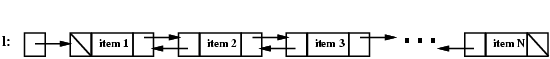Each node in a doubly linked list contains three fields: the data and two pointers. One pointer points to the previous node in the lis, and the other pointer points to the next node in the list. The previous pointer of the first node and the next pointer of the last node are both null. Here's the Java class definition for a doubly linked list node:

```public class DblListnode<E> {
//*** fields ***
private DblListnode<E> prev;
private E data;
private DblListnode<E> next;

//*** constructors ***
// 3 constructors
public DblListnode() {
this(null, null, null);
}

public DblListnode(E d) {
this(null, d, null);
}

public DblListnode(DblListnode<E> p, E d, DblListnode<E> n) {
prev = p;
data = d;
next = n;
}

//*** methods ***
public E getData() {
return data;
}

public DblListnode<E> getNext() {
return next;
}

public DblListnode<E> getPrev() {
return prev;
}

// modify fields
public void setData(E d) {
data = d;
}

public void setNext(DblListnode<E> n) {
next = n;
}

public void setPrev(DblListnode<E> p) {
prev = p;
}
}```

To remove a given node n from a doubly linked list, we need to change the prev field of the node to its right and we need to change the next field of the node to its left, as illustrated below.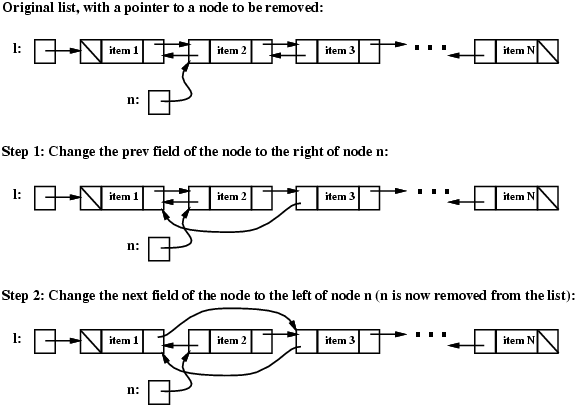Here's the code for removing node n:

```// Step 1: change the prev field of the node after n
DblListnode<E> tmp = n.getNext();
tmp.setPrev(n.getPrev());

// Step 2: change the next field of the node before n
tmp = n.getPrev();
tmp.setNext(n.getNext());```

Unfortunately, this code doesn't work (causes an attempt to dereference a null pointer) if n is either the first or the last node in the list. We can add code to test for these special cases, or we can use a circular, doubly linked list, as discussed below.

Both singly and doubly linked lists can be made circular. Here are the conceptual pictures: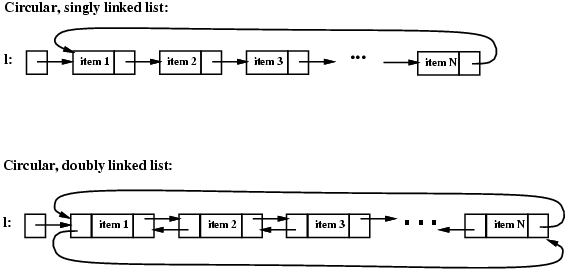The class definitions are the same as for the non-circular versions. The difference is that, instead of being null, the next field of the last node points to the first node and (for doubly linked circular lists) the prev field of the first node points to the last node.

The code given above for removing node n from a doubly linked list will work correctly except when node n is the first node in the list. In that case, the variable l that points to the first node in the list needs to be updated, so special-case code will always be needed unless the list includes a header node.

Another issue that you must address if you use a circular linked list is that if you're not careful, you may end up going round and round in circles! For example, what happens if you try to search for a particular value val using code like this:

```Listnode<E> tmp = L;
while (tmp != null && !tmp.getData().equals(val)) {
tmp = tmp.getNext();
}```

and the value is not in the list? You will have an infinite loop!

TEST YOURSELF #8

Write the correct code to search for value val in a circular linked list pointed to by l. (Assume that the list contains no null data values.)

With circular lists, you don't need pointers to both ends of the list; a pointer to one end suffices. With singly linked circular lists, it is most convenient to use only a pointer to the last node. With the structure it is easy to write code that does each of the following three operations in O(1) (constant) time: addFirst, addLast, removeFirst

TEST YOURSELF #9

Write the code for these operations. Assume you're adding these operations to a class named CircularLinkedList, which has a last reference to the last node in the circular singly linked list.

solution

### Comparison of Linked List Variations

The major disadvantage of doubly linked lists (over singly linked lists) is that they require more space (every node has two pointer fields instead of one). Also, the code to manipulate doubly linked lists needs to maintain the prev fields as well as the next fields; the more fields that have to be maintained, the more chance there is for errors.

The major advantage of doubly linked lists is that they make some operations (like the removal of a given node, or a right-to-left traversal of the list) more efficient.

The major advantage of circular lists (over non-circular lists) is that they eliminate some special-case code for some operations. Also, some applications lead naturally to circular list representations. For example, a computer network might best be modeled using a circular list.